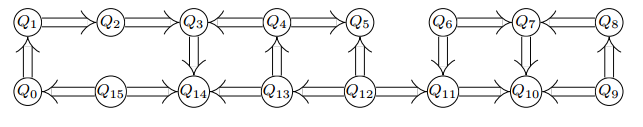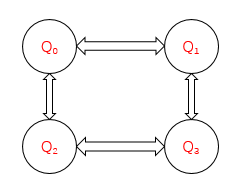# 获取量子线路对应矩阵¶

## 实例¶

#include "QPanda.h"
USING_QPANDA

int main()
{
auto qvm = initQuantumMachine(QMachineType::CPU);
auto q = qvm->qAllocMany(2);
auto c = qvm->cAllocMany(2);

// 构建量子线路
QCircuit cir;
cir << H(q)
<< CNOT(q, q)
<< H(q);

// 获取线路对应矩阵
QStat cir_matrix = getCircuitMatrix(cir);

// 打印矩阵信息
std::cout << cir_matrix << std::endl;

destroyQuantumMachine(qvm);
return 0;
}


1. 首先在主程序中用 initQuantumMachine() 初始化一个量子虚拟机对象，用于管理后续一系列行为
2. 接着用 qAllocMany() 和 cAllocMany()初始化量子比特与经典寄存器数目
3. 然后构建量子线路 cir
4. 最后调用接口 getCircuitMatrix() 输出量子线路的对应矩阵，并打印矩阵

# 判断量子逻辑门是否匹配量子拓扑结构¶## 接口介绍¶

isMatchTopology：判断量子逻辑门是否符合量子比特拓扑结构。第一个输入参数是目标量子逻辑门QGate，第二个输入参数是量子比特拓扑结构，返回值为布尔值，表示目标量子逻辑门是否满足量子比特拓扑结构。True为满足，False为不满足。

vector<vector<int>> qubits_topology = { {0,1,1,0},
{1,0,0,1},
{1,0,0,1},
{0,1,1,0} };

bool result = isMatchTopology(CNOT(q,q),qubits_topology);CNOT逻辑门操作的是1,3号量子比特，而从图中可以看出1,3号量子比特是相连的，所以得到的结果为true。

# 获得指定位置的量子逻辑门的相邻量子逻辑门¶

## 实例¶

#include "QPanda.h"
USING_QPANDA
int main(void)
{
auto qvm = initQuantumMachine(QMachineType::CPU);
auto q = qvm->qAllocMany(4);
auto c = qvm->cAllocMany(4);
QProg prog;

// 构建量子程序
prog << H(q)
<< H(q)
<< H(q)
<< H(q)
<< RX(q, PI / 2)
<< CNOT(q, q)
<< RX(q, PI / 2)
<< RX(q, PI / 2)
<< RX(q, PI / 2)
<< CNOT(q, q);

auto node_iter = prog.getFirstNodeIter();
std::vector<NodeInfo> node_iter_vector;

// 获取量子程序中node_iter位置上的的相邻节点

// 打印前后相邻节点类型
std::cout << "The previous node type : " << node_iter_vector.m_node_type << std::endl;
std::cout << "The node type that follows :" << node_iter_vector.m_node_type << std::endl;

destroyQuantumMachine(qvm);
return 0;
}


1. 构建一个量子程序prog；
2. 获取prog的第一个量子逻辑门的迭代器node_iter；

1. 目标量子逻辑门相邻量子逻辑门迭代器的集合永远包含两个元素；第一个元素是前一个量子逻辑门的迭代器，第二个元素是后一个量子逻辑门的迭代器。
2. 如果目标量子逻辑门是量子程序的第一个节点，那么输出的参数目标量子逻辑门相邻量子逻辑门迭代器的集合中则只能获取目标量子逻辑门后一个量子逻辑门的迭代器，集合的第一个元素为空迭代器。
3. 如果目标量子逻辑门是量子程序的最后一个量子逻辑门，那么输出的参数目标量子逻辑门相邻量子逻辑门迭代器的集合中则只能获取目标量子逻辑门前一个量子逻辑门的迭代器，集合的第二个元素为空迭代器。
4. 如果目标量子逻辑门前一个节点是QIf或者QWhile，那么输出的参数目标量子逻辑门相邻量子逻辑门迭代器的集合中则只能获取目标量子逻辑门后一个量子逻辑门的迭代器，集合的第一个元素为空迭代器。
5. 如果目标量子逻辑门后一个节点是QIf或者QWhile，那么输出的参数目标量子逻辑门相邻量子逻辑门迭代器的集合中则只能获取目标量子逻辑门前一个量子逻辑门的迭代器，集合的第二个元素为空迭代器。
6. 如果目标量子逻辑门是QWhile的第一个量子逻辑门，那么输出的参数目标量子逻辑门相邻量子逻辑门迭代器的集合中则只能获取目标量子逻辑门后一个量子逻辑门的迭代器，集合的第一个元素为空迭代器。
7. 如果目标量子逻辑门是QWhile的最后量子逻辑门，那么输出的参数目标量子逻辑门相邻量子逻辑门迭代器的集合中则只能获取目标量子逻辑门前一个量子逻辑门的迭代器，集合的第二个元素为空迭代器。

# 判断两个量子逻辑门是否可交换位置¶

## 实例¶

1. 构建一个量子程序prog；
2. 获取prog的第一个量子逻辑门的迭代器node_iter和最后一个量逻辑门的迭代器last_node_iter；
3. 调用isSwappable接口判断指定位置的两个逻辑门能否交换位置。
#include "QPanda.h"
USING_QPANDA
int main(void)
{
auto qvm = initQuantumMachine(QMachineType::CPU);
auto q = qvm->qAllocMany(4);
auto c = qvm->cAllocMany(4);
QProg prog;

// 构建量子程序
prog << H(q)
<< H(q)
<< H(q)
<< H(q)
<< RX(q, PI / 2)
<< CNOT(q, q)
<< RX(q, PI / 2)
<< RX(q, PI / 2)
<< RX(q, PI / 2)
<< CNOT(q, q);

// 获取待判断的节点
auto node_iter = prog.getFirstNodeIter();
auto last_node_iter = prog.getLastNodeIter();

// 判断节点是否可交换
bool result = isSwappable(prog, node_iter, last_node_iter);

if (result)
std::cout << "is  swappable! " << std::endl;
else
std::cout << "is not swappable! " << std::endl;

destroyQuantumMachine(qvm);
return 0;
}


# 判断逻辑门是否属于量子芯片支持的量子逻辑门集合¶

single_gates.push_back("RX");
single_gates.push_back("RY");
single_gates.push_back("RZ");
single_gates.push_back("X1");
single_gates.push_back("H");
single_gates.push_back("S");

double_gates.push_back("CNOT");
double_gates.push_back("CZ");
double_gates.push_back("ISWAP");


<QGate>
<SingleGate>
<Gate time = "2">rx</Gate>
<Gate time = "2">Ry</Gate>
<Gate time = "2">RZ</Gate>
<Gate time = "2">S</Gate>
<Gate time = "2">H</Gate>
<Gate time = "2">X1</Gate>
</SingleGate>
<DoubleGate>
<Gate time = "5">CNOT</Gate>
<Gate time = "5">CZ</Gate>
<Gate time = "5">ISWAP</Gate>
</DoubleGate>
</QGate>


#include "QPanda.h"
USING_QPANDA

int main(void)
{
auto qvm = initQuantumMachine();
auto q = qvm->qAllocMany(5);
QProg prog;

// 构建待判断的逻辑门
prog << H(q);

// 判断逻辑门类型是否支持
bool result = isSupportedGateType(prog.getFirstNodeIter());

if (result)
std::cout << "Gate type is supported !";
else
std::cout << "Gate type is not supported !";

destroyQuantumMachine(qvm);
return 0;
}